Support the Monkey! Tell All your Friends and TeachersHome MonkeyNotes Printable Notes Digital Library Study Guides Study Smart Parents Tips College Planning Test Prep Fun Zone Help / FAQ How to Cite Request a New Title

7.6 Conditional Probability

In many situations you get more information than simply the total outcomes and favorable outcomes you already have and, hence you are in position to make yourself more informed to make judgements regarding the probabilities of such situations. For example, suppose a card is drawn at random from a deck of 52 cards. Let B denotes the event the card is a diamond and A denotes the event the card is red. We may then consider the following probabilities.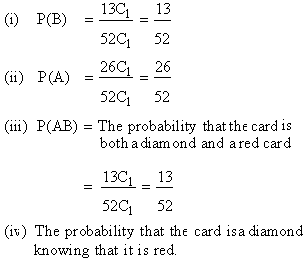Since there are 26 red cards of which 13 are diamonds, the probability that the card is diamond is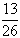. In other words the probability of event B knowing that A has occurred is.

The probability of B under the condition that A has occurred is known as condition probability and it is denoted by P (B/A) . Thus P (B/A) =. It should be observed that the probability of the event B is increased due to the additional information that the event A has occurred.

Conditional probability found using the formula P (B/A) =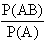Justification :- P (A/B) =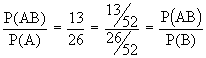Similarly P(A/B) =In both the cases if A and B are independent events then P (A/B) = P (A) and P(B/A) = P(B)
Therefore P(A) =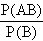Þ P (AB) = P (A) . P (B)
or P(B) =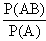Þ P (AB) = P(A) . P (B)Your browser does not support the IFRAME tag.

Propositions

(1) If A and B are independent events then A and B' are also independent where B' is the complementary event B.

(2) If A and B are independent events then A' and B' are also independent events.

(3) Two independent events cannot be mutually exclusive.

(4) De Morgans Laws : P (A È B)' = P (A' Ç B')

P (A Ç B)' = P (A' È B' )

Example A bag contains 6 red and 4 blue balls. One ball is drawn at random from the first bag and put into the second bag. A ball is then drawn from the second bag. What is the probability that is red ?

Solution :
Let A = getting a red ball from the 1st bag.
B = getting a blue from the first bag.
C = getting a red ball from the second bag after the ball drawn from the first bag is put into it .

Index

7. 1 Introduction
7.2 Trial
7.3 Sample Space
7. 4 Definition of Probability
7. 5 The Laws of Probability
7. 6 Conditional Probability
7. 7 Theoretical Distribution
7. 8 Binomial Distribution
7. 9 Normal Distribution
Chapter 8Search: All Products Books Popular Music Classical Music Video DVD Toys & Games Electronics Software Tools & Hardware Outdoor Living Kitchen & Housewares Camera & Photo Cell Phones Keywords: